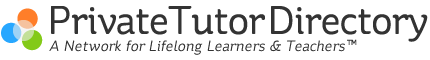Order of Operation
February 21, 2009 by Cindy  |

When you are usings the order of operations in Math, usually introduced in 6th grade there is a trick. Just remember

PEMDAS

P-parenthesis

E-exponents

M-multiply

D-divide

S- subtract

For younger grades remember MDAS.....  MY Dear Aunt Sally

M-multiply              My

D-divide                 Dear